# 嘿嘿，我就知道面試官接下來要問我 ConcurrentHashMap 底層原理了，看我怎么秀他

## 前言## 更正錯誤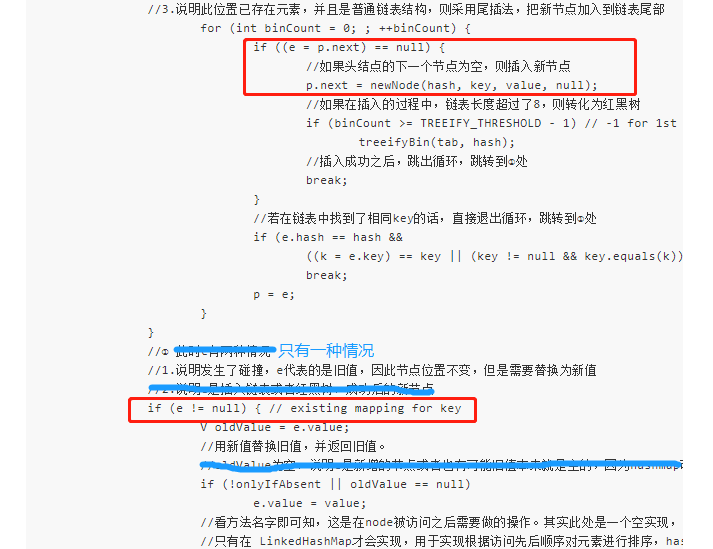put 方法，在新值替換舊值那里，應該是只有一種情況的，e 不包括新值。圖中的方框也標注出來了。因為，判斷 e=p.next==null ， 然后新的節點是賦值給 p.next 了，并沒有賦值給 e，此時 e 依舊是空的。所以 e!=null，代表當前的 e 是已經存在的舊值。

## 文章目錄

1）多線程下的 HashMap 有什么問題？

2）怎樣保證線程安全，為什么選用 ConcurrentHashMap？

3）ConcurrentHashMap 1.7 源碼解析

• 底層存儲結構
• 常用變量
• 構造函數
• put() 方法
• ensureSegment() 方法
• scanAndLockForPut() 方法
• rehash() 擴容機制
• get() 獲取元素方法
• remove() 方法
• size() 方法是怎么統計元素個數的

4）ConcurrentHashMap 1.8 源碼解析

• put()方法詳解
• initTable()初始化表
• transfer()是怎樣擴容和遷移元素的
• helpTransfer()方法幫助遷移元素

## 多線程下 HashMap 有什么問題？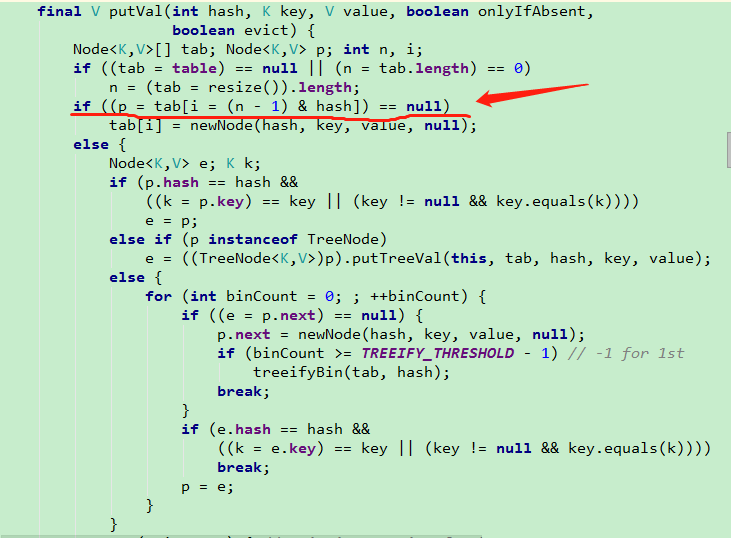## 怎樣保證線程安全，為什么選用 ConcurrentHashMap？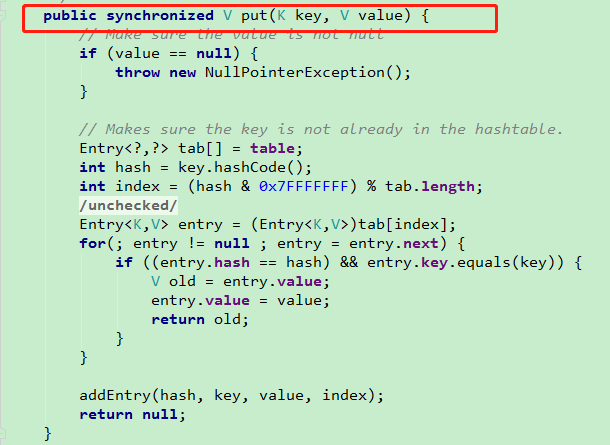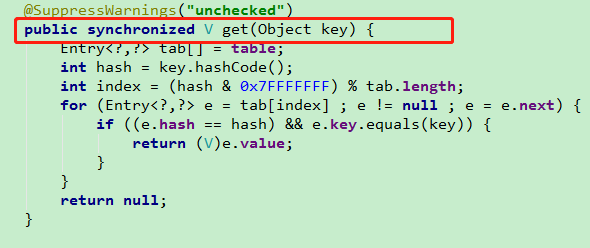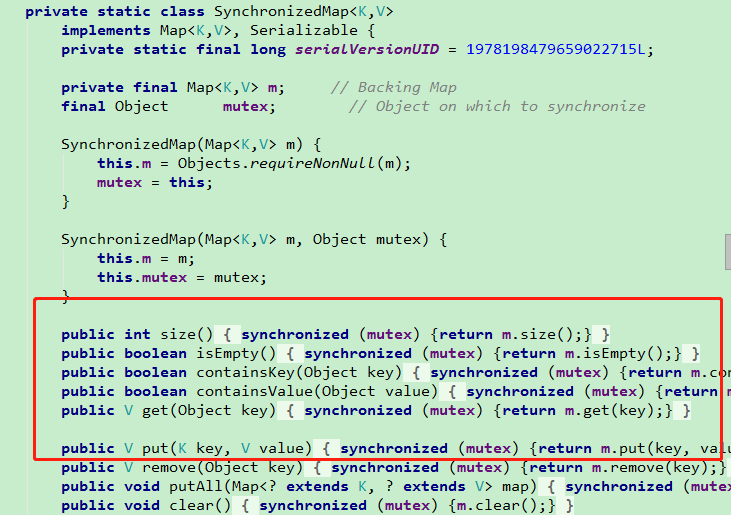## ConcurrentHashMap 1.7 源碼解析

### 底層存儲結構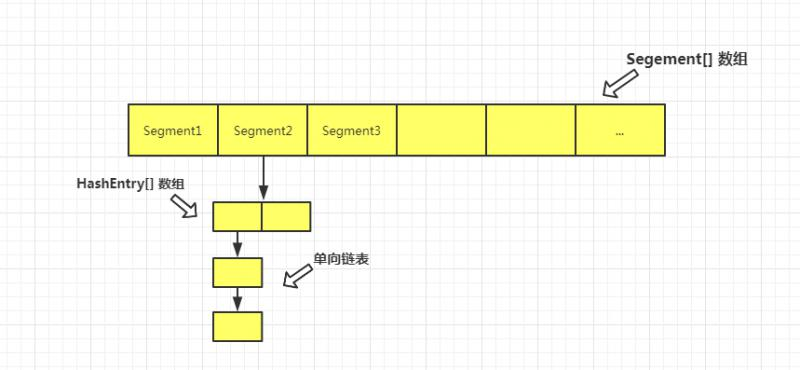### 常用變量

//默認初始化容量，這個和 HashMap中的容量是一個概念，表示的是整個 Map的容量
static final int DEFAULT_INITIAL_CAPACITY = 16;

//默認加載因子
static final float DEFAULT_LOAD_FACTOR = 0.75f;

//默認的并發級別，這個參數決定了 Segment 數組的長度
static final int DEFAULT_CONCURRENCY_LEVEL = 16;

//最大的容量
static final int MAXIMUM_CAPACITY = 1 << 30;

//每個Segment中table數組的最小長度為2，且必須是2的n次冪。
//由于每個Segment是懶加載的，用的時候才會初始化，因此為了避免使用時立即調整大小，設定了最小容量2
static final int MIN_SEGMENT_TABLE_CAPACITY = 2;

//用于限制Segment數量的最大值，必須是2的n次冪
static final int MAX_SEGMENTS = 1 << 16; // slightly conservative

//在size方法和containsValue方法，會優先采用樂觀的方式不加鎖，直到重試次數達到2，才會對所有Segment加鎖
//這個值的設定，是為了避免無限次的重試。后邊size方法會詳講怎么實現樂觀機制的。
static final int RETRIES_BEFORE_LOCK = 2;

//segment掩碼值，用于根據元素的hash值定位所在的 Segment 下標。后邊會細講

final int segmentShift;

// Segment 組成的數組，每一個 Segment 都可以看做是一個特殊的 HashMap
final Segment<K,V>[] segments;

//Segment 對象，繼承自 ReentrantLock 可重入鎖。
//其內部的屬性和方法和 HashMap 神似，只是多了一些拓展功能。
static final class Segment<K,V> extends ReentrantLock implements Serializable {

//這是在 scanAndLockForPut 方法中用到的一個參數，用于計算最大重試次數
//獲取當前可用的處理器的數量，若大于1，則返回64，否則返回1。
static final int MAX_SCAN_RETRIES =
Runtime.getRuntime().availableProcessors() > 1 ? 64 : 1;

//用于表示每個Segment中的 table，是一個用HashEntry組成的數組。
transient volatile HashEntry<K,V>[] table;

//Segment中的元素個數，每個Segment單獨計數（下邊的幾個參數同樣的都是單獨計數）
transient int count;

//每次 table 結構修改時，如put，remove等，此變量都會自增
transient int modCount;

//當前Segment擴容的閾值，同HashMap計算方法一樣也是容量乘以加載因子
//需要知道的是，每個Segment都是單獨處理擴容的，互相之間不會產生影響
transient int threshold;

//加載因子

//Segment構造函數
Segment(float lf, int threshold, HashEntry<K,V>[] tab) {
this.threshold = threshold;
this.table = tab;
}

...
// put(),remove(),rehash() 方法都在此類定義
}

// HashEntry，存在于每個Segment中，它就類似于HashMap中的Node，用于存儲鍵值對的具體數據和維護單向鏈表的關系
static final class HashEntry<K,V> {
//每個key通過哈希運算后的結果，用的是 Wang/Jenkins hash 的變種算法，此處不細講，感興趣的可自行查閱相關資料
final int hash;
final K key;
//value和next都用 volatile 修飾，用于保證內存可見性和禁止指令重排序
volatile V value;
//指向下一個節點
volatile HashEntry<K,V> next;

HashEntry(int hash, K key, V value, HashEntry<K,V> next) {
this.hash = hash;
this.key = key;
this.value = value;
this.next = next;
}
}


### 構造函數

ConcurrentHashMap 有五種構造函數，但是最終都會調用同一個構造函數，所以只需要搞明白這一個核心的構造函數就可以了。

PS: 文章注釋中 (1)(2)(3) 等序號都是用來方便做標記，不是計算值

public ConcurrentHashMap(int initialCapacity,
//檢驗參數是否合法。值得說的是，并發級別一定要大于0，否則就沒辦法實現分段鎖了。
if (!(loadFactor > 0) || initialCapacity < 0 || concurrencyLevel <= 0)
throw new IllegalArgumentException();
//并發級別不能超過最大值
if (concurrencyLevel > MAX_SEGMENTS)
concurrencyLevel = MAX_SEGMENTS;
// Find power-of-two sizes best matching arguments
//偏移量，是為了對hash值做位移操作，計算元素所在的Segment下標，put方法詳講
int sshift = 0;
//用于設定最終Segment數組的長度，必須是2的n次冪
int ssize = 1;
//這里就是計算 sshift 和 ssize 值的過程  (1)
while (ssize < concurrencyLevel) {
++sshift;
ssize <<= 1;
}
this.segmentShift = 32 - sshift;
//Segment的掩碼
if (initialCapacity > MAXIMUM_CAPACITY)
initialCapacity = MAXIMUM_CAPACITY;
//c用于輔助計算cap的值   (2)
int c = initialCapacity / ssize;
if (c * ssize < initialCapacity)
++c;
// cap 用于確定某個Segment的容量，即Segment中HashEntry數組的長度
int cap = MIN_SEGMENT_TABLE_CAPACITY;
//(3)
while (cap < c)
cap <<= 1;
// create segments and segments
//三個參數，創建一個Segment對象，保存到S0對象中。后邊在 ensureSegment 方法會用到S0作為原型對象去創建對應的Segment。
Segment<K,V> s0 =
(HashEntry<K,V>[])new HashEntry[cap]);
//創建出長度為 ssize 的一個 Segment數組
Segment<K,V>[] ss = (Segment<K,V>[])new Segment[ssize];
//把S0存到Segment數組中去。在這里，我們就可以發現，此時只是創建了一個Segment數組，
//但是并沒有把數組中的每個Segment對象創建出來，僅僅創建了一個Segment用來作為原型對象。
UNSAFE.putOrderedObject(ss, SBASE, s0); // ordered write of segments
this.segments = ss;
}


//假設調用了默認構造，都用的是默認參數，即 initialCapacity 和 concurrencyLevel 都是16
//(1)  sshift 和 ssize 值的計算過程為，每次循環，都會把 sshift 自增1，并且 ssize 左移一位，即乘以2，
//直到 ssize 的值大于等于 concurrencyLevel 的值 16。
sshfit=0,1,2,3,4
ssize=1,2,4,8,16
//可以看到，初始他們的值分別是0和1，最終結果是4和16
//sshfit是為了輔助計算segmentShift值，ssize是為了確定Segment數組長度。
//(2)  此時,計算c的值，
c = 16/16 = 1;
//判斷 c * 16 < 16 是否為真，真的話 c 自增1，此處為false，因此 c的值為1不變。
//(3)  此時，由于c為1， cap為2 ，因此判斷 cap < c 為false，最終cap為2。
//總結一下，以上三個步驟，最終都是為了確定以下幾個關鍵參數的值，
//確定 segmentShift ，這個用于后邊計算hash值的偏移量，此處即為 32-4=28，
//確定 ssize，必須是一個大于等于 concurrencyLevel 的一個2的n次冪值
//確定 cap，必須是一個大于等于2的一個2的n次冪值
//感興趣的小伙伴，還可以用另外幾組參數來計算上邊的參數值，可以加深理解參數的含義。
//例如initialCapacity和concurrencyLevel分別傳入10和5，或者傳入33和16


### put()方法

put 方法的總體流程是，

1. 通過哈希算法計算出當前 key 的 hash 值
2. 通過這個 hash 值找到它所對應的 Segment 數組的下標
3. 再通過 hash 值計算出它在對應 Segment 的 HashEntry數組 的下標
4. 找到合適的位置插入元素
//這是Map的put方法
public V put(K key, V value) {
Segment<K,V> s;
//不支持value為空
if (value == null)
throw new NullPointerException();
//通過 Wang/Jenkins 算法的一個變種算法，計算出當前key對應的hash值
int hash = hash(key);
//上邊我們計算出的 segmentShift為28，因此hash值右移28位，說明此時用的是hash的高4位，
//然后把它和掩碼15進行與運算，得到的值一定是一個 0000 ~ 1111 范圍內的值，即 0~15 。
int j = (hash >>> segmentShift) & segmentMask;
//這里是用Unsafe類的原子操作找到Segment數組中j下標的 Segment 對象
if ((s = (Segment<K,V>)UNSAFE.getObject          // nonvolatile; recheck
(segments, (j << SSHIFT) + SBASE)) == null) //  in ensureSegment
//初始化j下標的Segment
s = ensureSegment(j);
//在此Segment中添加元素
return s.put(key, hash, value, false);
}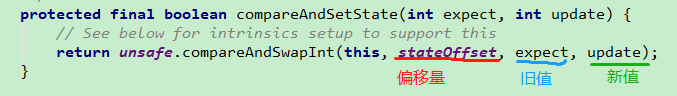(j << SSHIFT) + SBASE 就相當于圖中的 stateOffset偏移量。只不過圖中是 CAS 設置新值，而我們這里是取 j 的最新值。 后邊很多這樣的計算方式，就不贅述了。接著看 s.put 方法，這才是最終確定元素位置的方法。

//Segment中的 put 方法
final V put(K key, int hash, V value, boolean onlyIfAbsent) {
//這里通過tryLock嘗試加鎖，如果加鎖成功，返回null，否則執行 scanAndLockForPut方法
//這里說明一下，tryLock 和 lock 是 ReentrantLock 中的方法，
//區別是 tryLock 不會阻塞，搶鎖成功就返回true，失敗就立馬返回false，
//而 lock 方法是，搶鎖成功則返回，失敗則會進入同步隊列，阻塞等待獲取鎖。
HashEntry<K,V> node = tryLock() ? null :
scanAndLockForPut(key, hash, value);
V oldValue;
try {
//當前Segment的table數組
HashEntry<K,V>[] tab = table;
//這里就是通過hash值，與tab數組長度取模，找到其所在HashEntry數組的下標
int index = (tab.length - 1) & hash;
//當前下標位置的第一個HashEntry節點
HashEntry<K,V> first = entryAt(tab, index);
for (HashEntry<K,V> e = first;;) {
//如果第一個節點不為空
if (e != null) {
K k;
//并且第一個節點，就是要插入的節點，則替換value值，否則繼續向后查找
if ((k = e.key) == key ||
(e.hash == hash && key.equals(k))) {
//替換舊值
oldValue = e.value;
if (!onlyIfAbsent) {
e.value = value;
++modCount;
}
break;
}
e = e.next;
}
//說明當前index位置不存在任何節點，此時first為null，
//或者當前index存在一條鏈表，并且已經遍歷完了還沒找到相等的key，此時first就是鏈表第一個元素
else {
//如果node不為空，則直接頭插
if (node != null)
node.setNext(first);
//否則，創建一個新的node，并頭插
else
node = new HashEntry<K,V>(hash, key, value, first);
int c = count + 1;
//如果當前Segment中的元素大于閾值，并且tab長度沒有超過容量最大值，則擴容
if (c > threshold && tab.length < MAXIMUM_CAPACITY)
rehash(node);
//否則，就把當前node設置為index下標位置新的頭結點
else
setEntryAt(tab, index, node);
++modCount;
//更新count值
count = c;
//這種情況說明舊值肯定為空
oldValue = null;
break;
}
}
} finally {
//需要注意ReentrantLock必須手動解鎖
unlock();
}
//返回舊值
return oldValue;
}


//下邊的hash值是通過哈希運算后的hash值，不是hashCode
//計算 Segment 下標
//計算 HashEntry 數組下標
(tab.length - 1) & hash


//我們以并發級別16，HashEntry數組容量 4 為例，則它們參與運算的掩碼分別為 15 和 3
//hash值
0110 1101 0110 1111 0110 1110 0010 0010
0000 0000 0000 0000 0000 0000 0000 1111
//tab.length - 1 = 3     ，標記為 (2)
0000 0000 0000 0000 0000 0000 0000 0011
//用 hash 分別和 15 ，3 做與運算，會發現得到的結果是一樣，都是十進制 2.
//這表明，當前 hash值被分配到下標為 2 的 Segment 中，同時，被分配到下標為 2 的 HashEntry 數組中
//現在若有另外一個 hash 值 h2，和第一個hash值，高位不同，但是低4位相同，
1010 1101 0110 1111 0110 1110 0010 0010
//我們會發現，最后它也會被分配到下標為 2 的 Segment 和 HashEntry 數組，就會和第一個元素形成鏈表。
//所以，為了避免這種扎堆現象，讓元素盡量均勻分配，就讓 hash 的高 4 位和 (1)處做與 運算，而用低位和 (2)處做與運算
//這樣計算后，它們所在的Segment下標分別為 6(0110), 10(1010)，即使它們在HashEntry數組中的下標都為 2(0010)，也無所謂
//因為它們并不在一個 Segment 中，也就不會在同一個 HashEntry 數組中，更不會形成鏈表。
//更重要的是，它們不會有并發，因為在各自不同的 Segment 自己操作自己的加鎖解鎖，互不影響


put 方法比較簡單，只要能看懂 HashMap 中的 put 方法，這里也沒問題。主要是它調用的子方法比較復雜，下邊一個一個講解。

### ensureSegment()方法

//k為 (hash >>> segmentShift) & segmentMask 算法計算出來的值
private Segment<K,V> ensureSegment(int k) {
final Segment<K,V>[] ss = this.segments;
//u代表 k 的偏移量，用于通過 UNSAFE 獲取主內存最新的實際 K 值
long u = (k << SSHIFT) + SBASE; // raw offset
Segment<K,V> seg;
//從內存中取到最新的下標位置的 Segment 對象，判斷是否為空，(1)
if ((seg = (Segment<K,V>)UNSAFE.getObjectVolatile(ss, u)) == null) {
//之前構造函數說了，s0是作為一個原型對象，用于創建新的 Segment 對象
Segment<K,V> proto = ss; // use segment 0 as prototype
//容量
int cap = proto.table.length;
//加載因子
//擴容閾值
int threshold = (int)(cap * lf);
//把 Segment 對應的 HashEntry 數組先創建出來
HashEntry<K,V>[] tab = (HashEntry<K,V>[])new HashEntry[cap];
//再次檢查 K 下標位置的 Segment 是否為空， (2)
if ((seg = (Segment<K,V>)UNSAFE.getObjectVolatile(ss, u))
== null) { // recheck
//此處把 Segment 對象創建出來，并賦值給 s，
Segment<K,V> s = new Segment<K,V>(lf, threshold, tab);
//循環檢查 K 下標位置的 Segment 是否為空， (3)
//若不為空，則說明有其它線程搶先創建成功，并且已經成功同步到主內存中了，
//則把它取出來，并返回
while ((seg = (Segment<K,V>)UNSAFE.getObjectVolatile(ss, u))
== null) {
//CAS，若當前下標的Segment對象為空，就把它替換為最新創建出來的 s 對象。
//若成功，就跳出循環，否則，就一直自旋直到成功，或者 seg 不為空（其他線程成功導致）。
if (UNSAFE.compareAndSwapObject(ss, u, null, seg = s))
break;
}
}
}
return seg;
}


### scanAndLockForPut()方法

put 方法第一步搶鎖失敗之后，就會執行此方法，

private HashEntry<K,V> scanAndLockForPut(K key, int hash, V value) {
//根據hash值定位到它對應的HashEntry數組的下標位置，并找到鏈表的第一個節點
//注意，這個操作會從主內存中獲取到最新的狀態，以確保獲取到的first是最新值
HashEntry<K,V> first = entryForHash(this, hash);
HashEntry<K,V> e = first;
HashEntry<K,V> node = null;
//重試次數，初始化為 -1
int retries = -1; // negative while locating node
//若搶鎖失敗，就一直循環，直到成功獲取到鎖。有三種情況
while (!tryLock()) {
HashEntry<K,V> f; // to recheck first below
//1.若 retries 小于0，
if (retries < 0) {
if (e == null) {
//若 e 節點和 node 都為空，則創建一個 node 節點。這里只是預測性的創建一個node節點
if (node == null) // speculatively create node
node = new HashEntry<K,V>(hash, key, value, null);
retries = 0;
}
//如當前遍歷到的 e 節點不為空，則判斷它的key是否等于傳進來的key，若是則把 retries 設為0
else if (key.equals(e.key))
retries = 0;
//否則，繼續向后遍歷節點
else
e = e.next;
}
//2.若是重試次數超過了最大嘗試次數，則調用lock方法加鎖。表明不再重試，我下定決心了一定要獲取到鎖。
//要么當前線程可以獲取到鎖，要么獲取不到就去排隊等待獲取鎖。獲取成功后，再 break。
else if (++retries > MAX_SCAN_RETRIES) {
lock();
break;
}
//3.若 retries 的值為偶數，并且從內存中再次獲取到最新的頭節點，判斷若不等于first
//則說明有其他線程修改了當前下標位置的頭結點，于是需要更新頭結點信息。
else if ((retries & 1) == 0 &&
(f = entryForHash(this, hash)) != first) {
//更新頭結點信息，并把重試次數重置為 -1，繼續下一次循環，從最新的頭結點遍歷當前鏈表。
e = first = f; // re-traverse if entry changed
retries = -1;
}
}
return node;
}


Since traversal speed doesn't matter, we might as well help warm up the associated code and accesses as well.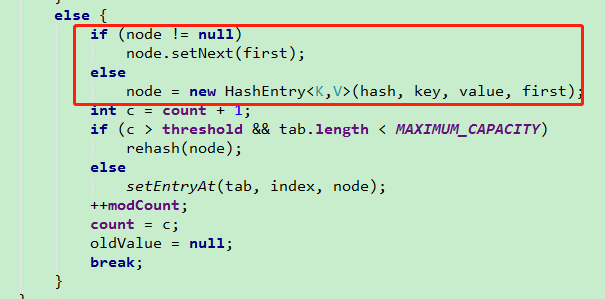scanAndLockForPut 這個方法可以確保返回時，當前線程一定是獲取到鎖的狀態。

### rehash()方法


//node為創建的新節點
private void rehash(HashEntry<K,V> node) {
//當前Segment中的舊表
HashEntry<K,V>[] oldTable = table;
//舊的容量
int oldCapacity = oldTable.length;
//新容量為舊容量的2倍
int newCapacity = oldCapacity << 1;
//更新新的閾值
//用新的容量創建一個新的 HashEntry 數組
HashEntry<K,V>[] newTable =
(HashEntry<K,V>[]) new HashEntry[newCapacity];
//當前的掩碼，用于計算節點在新數組中的下標
int sizeMask = newCapacity - 1;
//遍歷舊表
for (int i = 0; i < oldCapacity ; i++) {
HashEntry<K,V> e = oldTable[i];
//如果e不為空，說明當前鏈表不為空
if (e != null) {
HashEntry<K,V> next = e.next;
//計算hash值再新數組中的下標位置
int idx = e.hash & sizeMask;
//如果e不為空，且它的下一個節點為空，則說明這條鏈表只有一個節點，
//直接把這個節點放到新數組的對應下標位置即可
if (next == null)   //  Single node on list
newTable[idx] = e;
//否則，處理當前鏈表的節點遷移操作
else { // Reuse consecutive sequence at same slot
//記錄上一次遍歷到的節點
HashEntry<K,V> lastRun = e;
//對應上一次遍歷到的節點在新數組中的新下標
int lastIdx = idx;
for (HashEntry<K,V> last = next;
last != null;
last = last.next) {
//計算當前遍歷到的節點的新下標
int k = last.hash & sizeMask;
//若 k 不等于 lastIdx，則說明此次遍歷到的節點和上次遍歷到的節點不在同一個下標位置
//需要把 lastRun 和 lastIdx 更新為當前遍歷到的節點和下標值。
//若相同，則不處理，繼續下一次 for 循環。
if (k != lastIdx) {
lastIdx = k;
lastRun = last;
}
}
//把和 lastRun 節點的下標位置相同的鏈表最末尾的幾個連續的節點放到新數組的對應下標位置
newTable[lastIdx] = lastRun;
//再把剩余的節點，復制到新數組
//從舊數組的頭結點開始遍歷，直到 lastRun 節點，因為 lastRun節點后邊的節點都已經遷移完成了。
for (HashEntry<K,V> p = e; p != lastRun; p = p.next) {
V v = p.value;
int h = p.hash;
int k = h & sizeMask;
HashEntry<K,V> n = newTable[k];
//用的是復制節點信息的方式，并不是把原來的節點直接遷移，區別于lastRun處理方式
newTable[k] = new HashEntry<K,V>(h, p.key, v, n);
}
}
}
}
//所有節點都遷移完成之后，再處理傳進來的新的node節點，把它頭插到對應的下標位置
//頭插node節點
node.setNext(newTable[nodeIndex]);
newTable[nodeIndex] = node;
//更新當前Segment的table信息
table = newTable;
}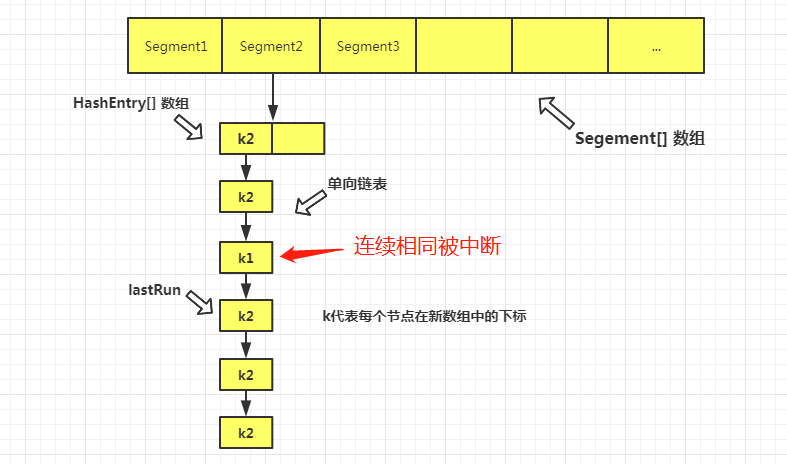（不知道為啥這個方法名起為 rehash，其實擴容時 hash 值并沒有重新計算，變化的只是它們所在的下標而已。我猜測，可能是，借用了 1.7 HashMap 中的說法吧。。。）

### get()

put 方法搞明白了之后，其實 get 方法就很好理解了。也是先定位到 Segment，然后再定位到 HashEntry 。

public V get(Object key) {
Segment<K,V> s; // manually integrate access methods to reduce overhead
HashEntry<K,V>[] tab;
//計算hash值
int h = hash(key);
//同樣的先定位到 key 所在的Segment ，然后從主內存中取出最新的節點
long u = (((h >>> segmentShift) & segmentMask) << SSHIFT) + SBASE;
if ((s = (Segment<K,V>)UNSAFE.getObjectVolatile(segments, u)) != null &&
(tab = s.table) != null) {
//若Segment不為空，且鏈表也不為空，則遍歷查找節點
for (HashEntry<K,V> e = (HashEntry<K,V>) UNSAFE.getObjectVolatile
(tab, ((long)(((tab.length - 1) & h)) << TSHIFT) + TBASE);
e != null; e = e.next) {
K k;
//找到則返回它的 value 值，否則返回 null
if ((k = e.key) == key || (e.hash == h && key.equals(k)))
return e.value;
}
}
return null;
}


### remove()

remove 方法和 put 方法類似，也不用做過多特殊的介紹，

public V remove(Object key) {
int hash = hash(key);
//定位到Segment
Segment<K,V> s = segmentForHash(hash);
//若 s為空，則返回 null，否則執行 remove
return s == null ? null : s.remove(key, hash, null);
}

public boolean remove(Object key, Object value) {
int hash = hash(key);
Segment<K,V> s;
return value != null && (s = segmentForHash(hash)) != null &&
s.remove(key, hash, value) != null;
}

final V remove(Object key, int hash, Object value) {
//嘗試加鎖，若失敗，則執行 scanAndLock ，此方法和 scanAndLockForPut 方法類似
if (!tryLock())
scanAndLock(key, hash);
V oldValue = null;
try {
HashEntry<K,V>[] tab = table;
int index = (tab.length - 1) & hash;
//從主內存中獲取對應 table 的最新的頭結點
HashEntry<K,V> e = entryAt(tab, index);
HashEntry<K,V> pred = null;
while (e != null) {
K k;
HashEntry<K,V> next = e.next;
//匹配到 key
if ((k = e.key) == key ||
(e.hash == hash && key.equals(k))) {
V v = e.value;
// value 為空，或者 value 也匹配成功
if (value == null || value == v || value.equals(v)) {
if (pred == null)
setEntryAt(tab, index, next);
else
pred.setNext(next);
++modCount;
--count;
oldValue = v;
}
break;
}
pred = e;
e = next;
}
} finally {
unlock();
}
return oldValue;
}


### size()

size 方法需要重點說明一下。愛思考的小伙伴可能就會想到，并發情況下，有可能在統計期間，數組元素個數不停的變化，而且，整個表還被分成了 N個 Segment，怎樣統計才能保證結果的準確性呢？ 我們一起來看下吧。

public int size() {
// Try a few times to get accurate count. On failure due to
// continuous async changes in table, resort to locking.
//segment數組
final Segment<K,V>[] segments = this.segments;
//統計所有Segment中元素的總個數
int size;
//如果size大小超過32位，則標記為溢出為true
boolean overflow;
//統計每個Segment中的 modcount 之和
long sum;
//上次記錄的 sum 值
long last = 0L;
//重試次數，初始化為 -1
int retries = -1;
try {
for (;;) {
//如果超過重試次數，則不再重試，而是把所有Segment都加鎖，再統計 size
if (retries++ == RETRIES_BEFORE_LOCK) {
for (int j = 0; j < segments.length; ++j)
//強制加鎖
ensureSegment(j).lock(); // force creation
}
sum = 0L;
size = 0;
overflow = false;
//遍歷所有Segment
for (int j = 0; j < segments.length; ++j) {
Segment<K,V> seg = segmentAt(segments, j);
//若當前遍歷到的Segment不為空，則統計它的 modCount 和 count 元素個數
if (seg != null) {
//累加當前Segment的結構修改次數，如put，remove等操作都會影響modCount
sum += seg.modCount;
int c = seg.count;
//若當前Segment的元素個數 c 小于0 或者 size 加上 c 的結果小于0，則認為溢出
//因為若超過了 int 最大值，就會返回負數
if (c < 0 || (size += c) < 0)
overflow = true;
}
}
//當此次嘗試，統計的 sum 值和上次統計的值相同，則說明這段時間內，
//并沒有任何一個 Segment 的結構發生改變，就可以返回最后的統計結果
if (sum == last)
break;
//不相等，則說明有 Segment 結構發生了改變，則記錄最新的結構變化次數之和 sum，
//并賦值給 last，用于下次重試的比較。
last = sum;
}
} finally {
//如果超過了指定重試次數，則說明表中的所有Segment都被加鎖了，因此需要把它們都解鎖
if (retries > RETRIES_BEFORE_LOCK) {
for (int j = 0; j < segments.length; ++j)
segmentAt(segments, j).unlock();
}
}
//若結果溢出，則返回 int 最大值，否則正常返回 size 值
return overflow ? Integer.MAX_VALUE : size;
}


## ConcurrentHashMap 1.8 源碼分析

PS： 由于1.8的 CHM 和 HashMap 結構和基本屬性變量，還有初始化邏輯都差不多，只是多了一些并發情況需要用到的參數和內部類，因此，不再單獨拎出來介紹。在方法中用到的時候，再詳細解釋。

### put()方法

public V put(K key, V value) {
return putVal(key, value, false);
}

final V putVal(K key, V value, boolean onlyIfAbsent) {
//可以看到，在并發情況下，key 和 value 都是不支持為空的。
if (key == null || value == null) throw new NullPointerException();
//這里和1.8 HashMap 的hash 方法大同小異，只是多了一個操作，如下
//( h ^ (h >>> 16)) & HASH_BITS;  HASH_BITS = 0x7fffffff;
// 0x7fffffff ，二進制為 0111 1111 1111 1111 1111 1111 1111 1111 。
//所以，hash值除了做了高低位異或運算，還多了一步，保證最高位的 1 個 bit 位總是0。
//這里，我并沒有明白它的意圖，僅僅是保證計算出來的hash值不超過 Integer 最大值，且不為負數嗎。
//同 HashMap 的hash 方法對比一下，會發現連源碼注釋都是相同的，并沒有多說明其它的。
//我個人認為意義不大，因為最后 hash 是為了和 capacity -1 做與運算，而 capacity 最大值為 1<<30，
//即 0100 0000 0000 0000 0000 0000 0000 0000 ，減1為 0011 1111 1111 1111 1111 1111 1111 1111。
//即使 hash 最高位為 1(無所謂0)，也不影響最后的結果，最高位也總會是0.
//用來計算當前鏈表上的元素個數
int binCount = 0;
for (Node<K,V>[] tab = table;;) {
Node<K,V> f; int n, i, fh;
//如果表為空，則說明還未初始化。
if (tab == null || (n = tab.length) == 0)
//初始化表，只有一個線程可以初始化成功。
tab = initTable();
//若表已經初始化，則找到當前 key 所在的桶，并且判斷是否為空
else if ((f = tabAt(tab, i = (n - 1) & hash)) == null) {
//若當前桶為空，則通過 CAS 原子操作，把新節點插入到此位置，
//這保證了只有一個線程可以 CAS 成功，其它線程都會失敗。
if (casTabAt(tab, i, null,
new Node<K,V>(hash, key, value, null)))
break;                   // no lock when adding to empty bin
}
//若所在桶不為空，則判斷節點的 hash 值是否為 MOVED（值是-1）
else if ((fh = f.hash) == MOVED)
//若為-1，說明當前數組正在進行擴容，則需要當前線程幫忙遷移數據
tab = helpTransfer(tab, f);
else {
V oldVal = null;
//這里用加同步鎖的方式，來保證線程安全，給桶中第一個節點對象加鎖
synchronized (f) {
//recheck 一下，保證當前桶的第一個節點無變化，后邊很多這樣類似的操作，不再贅述
if (tabAt(tab, i) == f) {
//如果hash值大于等于0，說明是正常的鏈表結構
if (fh >= 0) {
binCount = 1;
//從頭結點開始遍歷，每遍歷一次，binCount計數加1
for (Node<K,V> e = f;; ++binCount) {
K ek;
//如果找到了和當前 key 相同的節點，則用新值替換舊值
if (e.hash == hash &&
((ek = e.key) == key ||
(ek != null && key.equals(ek)))) {
oldVal = e.val;
if (!onlyIfAbsent)
e.val = value;
break;
}
Node<K,V> pred = e;
//若遍歷到了尾結點，則把新節點尾插進去
if ((e = e.next) == null) {
pred.next = new Node<K,V>(hash, key,
value, null);
break;
}
}
}
//否則判斷是否是樹節點。這里提一下，TreeBin只是頭結點對TreeNode的再封裝
else if (f instanceof TreeBin) {
Node<K,V> p;
binCount = 2;
if ((p = ((TreeBin<K,V>)f).putTreeVal(hash, key,
value)) != null) {
oldVal = p.val;
if (!onlyIfAbsent)
p.val = value;
}
}
}
}
//注意下，這個判斷是在同步鎖外部，因為 treeifyBin內部也有同步鎖，并不影響
if (binCount != 0) {
//如果節點個數大于等于 8，則轉化為紅黑樹
if (binCount >= TREEIFY_THRESHOLD)
treeifyBin(tab, i);
//把舊節點值返回
if (oldVal != null)
return oldVal;
break;
}
}
}
//給元素個數加 1，并有可能會觸發擴容，比較復雜，稍后細講
return null;
}


### initTable()方法

private final Node<K,V>[] initTable() {
Node<K,V>[] tab; int sc;
//循環判斷表是否為空，直到初始化成功為止。
while ((tab = table) == null || tab.length == 0) {
//sizeCtl 這個值有很多情況，默認值為0，
//當為 -1 時，說明有其它線程正在對表進行初始化操作
//當表初始化成功后，又會把它設置為擴容閾值
//當為一個小于 -1 的負數，用來表示當前有幾個線程正在幫助擴容(后邊細講)
if ((sc = sizeCtl) < 0)
//若 sc 小于0，其實在這里就是-1，因為此時表是空的，不會發生擴容，sc只能為正數或者-1
//因此，當前線程放棄 CPU 時間片，只是自旋。
Thread.yield(); // lost initialization race; just spin
//通過 CAS 把 sc 的值設置為-1，表明當前線程正在進行表的初始化，其它失敗的線程就會自旋
else if (U.compareAndSwapInt(this, SIZECTL, sc, -1)) {
try {
//重新檢查一下表是否為空
if ((tab = table) == null || tab.length == 0) {
//如果sc大于0，則為sc，否則返回默認容量 16。
//當調用有參構造創建 Map 時，sc的值是大于0的。
int n = (sc > 0) ? sc : DEFAULT_CAPACITY;
@SuppressWarnings("unchecked")
//創建數組
Node<K,V>[] nt = (Node<K,V>[])new Node<?,?>[n];
table = tab = nt;
//n減去 1/4 n ，即為 0.75n ，表示擴容閾值
sc = n - (n >>> 2);
}
} finally {
//更新 sizeCtl 為擴容閾值
sizeCtl = sc;
}
//若當前線程初始化表成功，則跳出循環。其它自旋的線程因為判斷數組不為空，也會停止自旋
break;
}
}
return tab;
}


CounterCell 會組成一個數組，也會涉及到擴容問題。所以，先畫一個示意圖幫助理解一下。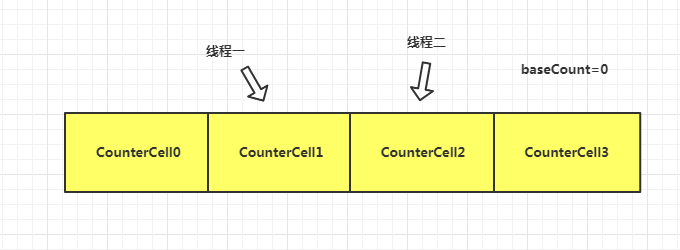//線程被分配到的格子
@sun.misc.Contended static final class CounterCell {
//此格子內記錄的 value 值
volatile long value;
CounterCell(long x) { value = x; }
}

//用來存儲線程和線程生成的隨機數的對應關系
static final int getProbe() {
}

// x為1,check代表鏈表上的元素個數
private final void addCount(long x, int check) {
CounterCell[] as; long b, s;
//此處要進入if有兩種情況
//1.數組不為空，說明數組已經被創建好了。
//2.若數組為空，說明數組還未創建，很有可能競爭的線程非常少，因此就直接 CAS 操作 baseCount
//若 CAS 成功，則方法跳轉到 (2)處，若失敗，則需要考慮給當前線程分配一個格子（指CounterCell對象）
if ((as = counterCells) != null ||
!U.compareAndSwapLong(this, BASECOUNT, b = baseCount, s = b + x)) {
CounterCell a; long v; int m;
//字面意思，是無競爭，這里先標記為 true，表示還沒有產生線程競爭
boolean uncontended = true;
//1.若數組為空，進方法 (1)
//然后用隨機數與數組長度取模，計算它所在的格子。若當前線程所分配到的格子為空，進方法 (1)。
//3.若數組不為空，且線程所在格子不為空，則嘗試 CAS 修改此格子對應的 value 值加1。
//若修改成功，則跳轉到 (3)，若失敗，則把 uncontended 值設為 fasle，說明產生了競爭，然后進方法 (1)
if (as == null || (m = as.length - 1) < 0 ||
(a = as[ThreadLocalRandom.getProbe() & m]) == null ||
!(uncontended =
U.compareAndSwapLong(a, CELLVALUE, v = a.value, v + x))) {
//方法(1), 這個方法的目的是讓當前線程一定把 1 加成功。情況更多，更復雜，稍后講。
return;
}
//(3)能走到這，說明數組不為空，且修改 baseCount失敗，
//且線程被分配到的格子不為空，且修改 value 成功。
//但是這里沒明白為什么小于等于1，就直接返回了，這里我懷疑之前的方法漏掉了binCount=0的情況。
//而且此處若返回了，后邊怎么判斷擴容？（存疑）
if (check <= 1)
return;
//計算總共的元素個數
s = sumCount();
}
//(2)這里用于檢查是否需要擴容（下邊這部分很多邏輯不懂的話，等后邊講完擴容，再回來看就理解了）
if (check >= 0) {
Node<K,V>[] tab, nt; int n, sc;
//若元素個數達到擴容閾值，且tab不為空，且tab數組長度小于最大容量
while (s >= (long)(sc = sizeCtl) && (tab = table) != null &&
(n = tab.length) < MAXIMUM_CAPACITY) {
//這里假設數組長度n就為16，這個方法返回的是一個固定值，用于當做一個擴容的校驗標識
//可以跳轉到最后，看詳細計算過程，0000 0000 0000 0000 1000 0000 0001 1011
int rs = resizeStamp(n);
//若sc小于0，說明正在擴容
if (sc < 0) {
//sc的結構類似這樣，1000 0000 0001 1011 0000 0000 0000 0001
//sc的高16位是數據校驗標識，低16位代表當前有幾個線程正在幫助擴容,RESIZE_STAMP_SHIFT=16
//因此判斷校驗標識是否相等，不相等則退出循環
//sc == rs + 1,sc == rs + MAX_RESIZERS 這兩個應該是用來判斷擴容是否已經完成，但是計算方法存疑
//感興趣的可以看這個地址，應該是一個 bug ，
// https://bugs.java.com/bugdatabase/view_bug.do?bug_id=JDK-8214427
//nextTable=null 說明需要擴容的新數組還未創建完成
//transferIndex這個參數小于等于0，說明已經不需要其它線程幫助擴容了，
//但是并不說明已經擴容完成，因為有可能還有線程正在遷移元素。稍后擴容細講就明白了。
if ((sc >>> RESIZE_STAMP_SHIFT) != rs || sc == rs + 1 ||
sc == rs + MAX_RESIZERS || (nt = nextTable) == null ||
transferIndex <= 0)
break;
//到這里說明當前線程可以幫助擴容，因此sc值加一，代表擴容的線程數加1
if (U.compareAndSwapInt(this, SIZECTL, sc, sc + 1))
transfer(tab, nt);
}
//當sc大于0，說明sc代表擴容閾值，因此第一次擴容之前肯定走這個分支，用于初始化新表 nextTable
//rs<<16
//1000 0000 0001 1011 0000 0000 0000 0000
//+2
//1000 0000 0001 1011 0000 0000 0000 0010
//這個值，轉為十進制就是 -2145714174，用于標識，這是擴容時，初始化新表的狀態，
//擴容時，需要用到這個參數校驗是否所有線程都全部幫助擴容完成。
else if (U.compareAndSwapInt(this, SIZECTL, sc,
(rs << RESIZE_STAMP_SHIFT) + 2))
//擴容，第二個參數代表新表，傳入null，則說明是第一次初始化新表(nextTable)
transfer(tab, null);
s = sumCount();
}
}
}

//計算表中的元素總個數
final long sumCount() {
CounterCell[] as = counterCells; CounterCell a;
//baseCount，以這個值作為累加基準
long sum = baseCount;
if (as != null) {
//遍歷 counterCells 數組，得到每個對象中的value值
for (int i = 0; i < as.length; ++i) {
if ((a = as[i]) != null)
//累加 value 值
sum += a.value;
}
}
//此時得到的就是元素總個數
return sum;
}

//擴容時的校驗標識
static final int resizeStamp(int n) {
return Integer.numberOfLeadingZeros(n) | (1 << (RESIZE_STAMP_BITS - 1));
}

//n=16，
0000 0000 0000 0000 0000 0000 0001 0000
//前面有27個0，即27
0000 0000 0000 0000 0000 0000 0001 1011
//RESIZE_STAMP_BITS為16，然后 1<<(16-1)，即 1<<15
0000 0000 0000 0000 1000 0000 0000 0000
//它們做或運算，得到 rs 的值
0000 0000 0000 0000 1000 0000 0001 1011


//傳過來的參數分別為 1 ， false
private final void fullAddCount(long x, boolean wasUncontended) {
int h;
//如果當前線程的隨機數為0，則強制初始化一個值
if ((h = ThreadLocalRandom.getProbe()) == 0) {
//此時把 wasUncontended 設為true，認為無競爭
wasUncontended = true;
}
//用來表示比 contend（競爭）更嚴重的碰撞，若為true，表示可能需要擴容，以減少碰撞沖突
boolean collide = false;                // True if last slot nonempty
//循環內，外層if判斷分三種情況，內層判斷又分為六種情況
for (;;) {
CounterCell[] as; CounterCell a; int n; long v;
//1. 若counterCells數組不為空。  建議先看下邊的2和3兩種情況，再回頭看這個。
if ((as = counterCells) != null && (n = as.length) > 0) {
// (1) 若當前線程所在的格子（CounterCell對象）為空
if ((a = as[(n - 1) & h]) == null) {
if (cellsBusy == 0) {
//若無鎖，則樂觀的創建一個 CounterCell 對象。
CounterCell r = new CounterCell(x);
//嘗試加鎖
if (cellsBusy == 0 &&
U.compareAndSwapInt(this, CELLSBUSY, 0, 1)) {
boolean created = false;
//加鎖成功后，再 recheck 一下數組是否不為空，且當前格子為空
try {
CounterCell[] rs; int m, j;
if ((rs = counterCells) != null &&
(m = rs.length) > 0 &&
rs[j = (m - 1) & h] == null) {
//把新創建的對象賦值給當前格子
rs[j] = r;
created = true;
}
} finally {
//手動釋放鎖
cellsBusy = 0;
}
//若當前格子創建成功，且上邊的賦值成功，則說明加1成功，退出循環
if (created)
break;
//否則，繼續下次循環
continue;           // Slot is now non-empty
}
}
//若cellsBusy=1，說明有其它線程搶鎖成功。或者若搶鎖的 CAS 操作失敗，都會走到這里，
//則當前線程需跳轉到(9)重新生成隨機數，進行下次循環判斷。
collide = false;
}
/**
*后邊這幾種情況，都是數組和當前隨機到的格子都不為空的情況。
*且注意每種情況，若執行成功，且不break，continue，則都會執行(9)，重新生成隨機數，進入下次循環判斷
*/
// (2) 到這，說明當前方法在被調用之前已經 CAS 失敗過一次，若不明白可回頭看下 addCount 方法，
//為了減少競爭，則跳轉到⑨處重新生成隨機數，并把 wasUncontended 設置為true ，認為下一次不會產生競爭
else if (!wasUncontended)       // CAS already known to fail
wasUncontended = true;      // Continue after rehash
// (3) 若 wasUncontended 為 true 無競爭，則嘗試一次 CAS。若成功，則結束循環，若失敗則判斷后邊的 (4)(5)(6)。
else if (U.compareAndSwapLong(a, CELLVALUE, v = a.value, v + x))
break;
// (4) 結合 (6) 一起看，(4)(5)(6)都是 wasUncontended=true，且CAS修改value失敗的情況。
//若數組有變化，或者數組長度大于等于當前CPU的核心數，則把 collide 改為 false
//因為數組若有變化，說明是由擴容引起的；長度超限，則說明已經無法擴容，只能認為無碰撞。
//這里很有意思，認真思考一下，當擴容超限后，則會達到一個平衡，即 (4)(5) 反復執行，直到 (3) 中CAS成功，跳出循環。
else if (counterCells != as || n >= NCPU)
collide = false;            // At max size or stale
// (5) 若數組無變化，且數組長度小于CPU核心數時，且 collide 為 false，就把它改為 true，說明下次循環可能需要擴容
else if (!collide)
collide = true;
// (6) 若數組無變化，且數組長度小于CPU核心數時，且 collide 為 true，說明沖突比較嚴重，需要擴容了。
else if (cellsBusy == 0 &&
U.compareAndSwapInt(this, CELLSBUSY, 0, 1)) {
try {
//recheck
if (counterCells == as) {// Expand table unless stale
//創建一個容量為原來兩倍的數組
CounterCell[] rs = new CounterCell[n << 1];
//轉移舊數組的值
for (int i = 0; i < n; ++i)
rs[i] = as[i];
//更新數組
counterCells = rs;
}
} finally {
cellsBusy = 0;
}
//認為擴容后，下次不會產生沖突了，和(4)處邏輯照應
collide = false;
//當次擴容后，就不需要重新生成隨機數了
continue;                   // Retry with expanded table
}
// (9)，重新生成一個隨機數，進行下一次循環判斷
}
//2.這里的 cellsBusy 參數非常有意思，是一個volatile的 int值，用來表示自旋鎖的標志，
//可以類比 AQS 中的 state 參數，用來控制鎖之間的競爭，并且是獨占模式。簡化版的AQS。
//cellsBusy 若為0，說明無鎖，線程都可以搶鎖，若為1，表示已經有線程拿到了鎖，則其它線程不能搶鎖。
else if (cellsBusy == 0 && counterCells == as &&
U.compareAndSwapInt(this, CELLSBUSY, 0, 1)) {
boolean init = false;
try {
//這里再重新檢測下 counterCells 數組引用是否有變化
if (counterCells == as) {
//初始化一個長度為 2 的數組
CounterCell[] rs = new CounterCell;
//根據當前線程的隨機數值，計算下標，只有兩個結果 0 或 1，并初始化對象
rs[h & 1] = new CounterCell(x);
//更新數組引用
counterCells = rs;
//初始化成功的標志
init = true;
}
} finally {
//別忘了，需要手動解鎖。
cellsBusy = 0;
}
//若初始化成功，則說明當前加1的操作也已經完成了，則退出整個循環。
if (init)
break;
}
//3.到這，說明數組為空，且 2 搶鎖失敗，則嘗試直接去修改 baseCount 的值，
//若成功，也說明加1操作成功，則退出循環。
else if (U.compareAndSwapLong(this, BASECOUNT, v = baseCount, v + x))
break;                          // Fall back on using base
}
}


### transfer()方法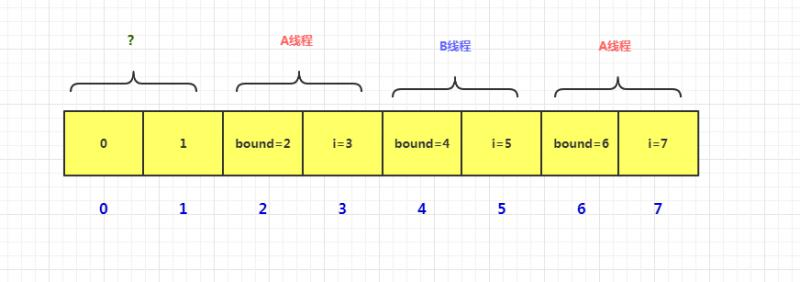//這個類是一個標志，用來代表當前桶（數組中的某個下標位置）的元素已經全部遷移完成
static final class ForwardingNode<K,V> extends Node<K,V> {
final Node<K,V>[] nextTable;
ForwardingNode(Node<K,V>[] tab) {
//把當前桶的頭結點的 hash 值設置為 -1，表明已經遷移完成，
//這個節點中并不存儲有效的數據
super(MOVED, null, null, null);
this.nextTable = tab;
}
}

//遷移數據
private final void transfer(Node<K,V>[] tab, Node<K,V>[] nextTab) {
int n = tab.length, stride;
//根據當前CPU核心數，確定每次推進的步長，最小值為16.（為了方便我們以2為例）
if ((stride = (NCPU > 1) ? (n >>> 3) / NCPU : n) < MIN_TRANSFER_STRIDE)
stride = MIN_TRANSFER_STRIDE; // subdivide range
if (nextTab == null) {            // initiating
try {
@SuppressWarnings("unchecked")
//新數組長度為原數組的兩倍
Node<K,V>[] nt = (Node<K,V>[])new Node<?,?>[n << 1];
nextTab = nt;
} catch (Throwable ex) {      // try to cope with OOME
sizeCtl = Integer.MAX_VALUE;
return;
}
//用 nextTable 指代新數組
nextTable = nextTab;
//這里就把推進的下標值初始化為原數組長度（以16為例）
transferIndex = n;
}
//新數組長度
int nextn = nextTab.length;
//創建一個標志類
ForwardingNode<K,V> fwd = new ForwardingNode<K,V>(nextTab);
//是否向前推進的標志
//是否所有線程都全部遷移完成的標志
boolean finishing = false; // to ensure sweep before committing nextTab
//i 代表當前線程正在遷移的桶的下標，bound代表它本次可以遷移的范圍下限
for (int i = 0, bound = 0;;) {
Node<K,V> f; int fh;
//需要向前推進
int nextIndex, nextBound;
// (1) 先看 (3) 。i每次自減 1，直到 bound。若超過bound范圍，或者finishing標志為true，則不用向前推進。
//若未全部完成遷移，且 i 并未走到 bound，則跳轉到 (7)，處理當前桶的元素遷移。
if (--i >= bound || finishing)
// (2) 每次執行，都會把 transferIndex 最新的值同步給 nextIndex
//若 transferIndex小于等于0，則說明原數組中的每個桶位置，都有線程在處理遷移了，
//于是，需要跳出while循環，并把 i設為 -1，以跳轉到④判斷在處理的線程是否已經全部完成。
else if ((nextIndex = transferIndex) <= 0) {
i = -1;
}
// (3) 第一個線程會先走到這里，確定它的數據遷移范圍。(2)處會更新 nextIndex為 transferIndex 的最新值
//因此第一次 nextIndex=n=16，nextBound代表當次遷移的數據范圍下限，減去步長即可，
//所以，第一次時，nextIndex=16，nextBound=16-2=14。后續，每次都會間隔一個步長。
else if (U.compareAndSwapInt
(this, TRANSFERINDEX, nextIndex,
nextBound = (nextIndex > stride ?
nextIndex - stride : 0))) {
//bound代表當次數據遷移下限
bound = nextBound;
//第一次的i為15，因為長度16的數組，最后一個元素的下標為15
i = nextIndex - 1;
//表明不需要向前推進，只有當把當前范圍內的數據全部遷移完成后，才可以向前推進
}
}
// (4)
if (i < 0 || i >= n || i + n >= nextn) {
int sc;
//若全部線程遷移完成
if (finishing) {
nextTable = null;
//更新table為新表
table = nextTab;
//擴容閾值改為原來數組長度的 3/2 ，即新長度的 3/4，也就是新數組長度的0.75倍
sizeCtl = (n << 1) - (n >>> 1);
return;
}
//到這，說明當前線程已經完成了自己的所有遷移（無論參與了幾次遷移），
//則把 sc 減1，表明參與擴容的線程數減少 1。
if (U.compareAndSwapInt(this, SIZECTL, sc = sizeCtl, sc - 1)) {
//在 addCount 方法最后，我們強調，遷移開始時，會設置 sc=(rs << RESIZE_STAMP_SHIFT) + 2
//每當有一個線程參與遷移，sc 就會加 1，每當有一個線程完成遷移，sc 就會減 1。
//因此，這里就是去校驗當前 sc 是否和初始值是否相等。相等，則說明全部線程遷移完成。
if ((sc - 2) != resizeStamp(n) << RESIZE_STAMP_SHIFT)
return;
//只有此處，才會把finishing 設置為true。
//這里非常有意思，會把 i 從 -1 修改為16，
//目的就是，讓 i 再從后向前掃描一遍數組，檢查是否所有的桶都已被遷移完成，參看 (6)
i = n; // recheck before commit
}
}
// (5) 若i的位置元素為空，則說明當前桶的元素已經被遷移完成，就把頭結點設置為fwd標志。
else if ((f = tabAt(tab, i)) == null)
advance = casTabAt(tab, i, null, fwd);
// (6) 若當前桶的頭結點是 ForwardingNode ，說明遷移完成，則向前推進
else if ((fh = f.hash) == MOVED)
//(7) 處理當前桶的數據遷移。
else {
synchronized (f) {  //給頭結點加鎖
if (tabAt(tab, i) == f) {
Node<K,V> ln, hn;
//若hash值大于等于0，則說明是普通鏈表節點
if (fh >= 0) {
int runBit = fh & n;
//這里是 1.7 的 CHM 的 rehash 方法和 1.8 HashMap的 resize 方法的結合體。
//會分成兩條鏈表，一條鏈表和原來的下標相同，另一條鏈表是原來的下標加數組長度的位置
//然后找到 lastRun 節點，從它到尾結點整體遷移。
//lastRun前邊的節點則單個遷移，但是需要注意的是，這里是頭插法。
//另外還有一點和1.7不同，1.7 lastRun前邊的節點是復制過去的，而這里是直接遷移的，沒有復制操作。
//所以，最后會有兩條鏈表，一條鏈表從 lastRun到尾結點是正序的，而lastRun之前的元素是倒序的，
//另外一條鏈表，從頭結點開始就是倒敘的。看下圖。
Node<K,V> lastRun = f;
for (Node<K,V> p = f.next; p != null; p = p.next) {
int b = p.hash & n;
if (b != runBit) {
runBit = b;
lastRun = p;
}
}
if (runBit == 0) {
ln = lastRun;
hn = null;
}
else {
hn = lastRun;
ln = null;
}
for (Node<K,V> p = f; p != lastRun; p = p.next) {
int ph = p.hash; K pk = p.key; V pv = p.val;
if ((ph & n) == 0)
ln = new Node<K,V>(ph, pk, pv, ln);
else
hn = new Node<K,V>(ph, pk, pv, hn);
}
setTabAt(nextTab, i, ln);
setTabAt(nextTab, i + n, hn);
setTabAt(tab, i, fwd);
}
//樹節點
else if (f instanceof TreeBin) {
TreeBin<K,V> t = (TreeBin<K,V>)f;
TreeNode<K,V> lo = null, loTail = null;
TreeNode<K,V> hi = null, hiTail = null;
int lc = 0, hc = 0;
for (Node<K,V> e = t.first; e != null; e = e.next) {
int h = e.hash;
TreeNode<K,V> p = new TreeNode<K,V>
(h, e.key, e.val, null, null);
if ((h & n) == 0) {
if ((p.prev = loTail) == null)
lo = p;
else
loTail.next = p;
loTail = p;
++lc;
}
else {
if ((p.prev = hiTail) == null)
hi = p;
else
hiTail.next = p;
hiTail = p;
++hc;
}
}
ln = (lc <= UNTREEIFY_THRESHOLD) ? untreeify(lo) :
(hc != 0) ? new TreeBin<K,V>(lo) : t;
hn = (hc <= UNTREEIFY_THRESHOLD) ? untreeify(hi) :
(lc != 0) ? new TreeBin<K,V>(hi) : t;
setTabAt(nextTab, i, ln);
setTabAt(nextTab, i + n, hn);
setTabAt(tab, i, fwd);
}
}
}
}
}
}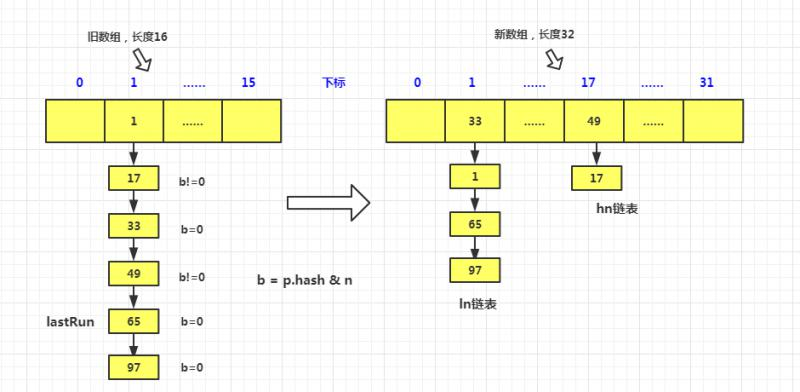### helpTransfer()方法

final Node<K,V>[] helpTransfer(Node<K,V>[] tab, Node<K,V> f) {
Node<K,V>[] nextTab; int sc;
//頭結點為 ForwardingNode ，并且新數組已經初始化
if (tab != null && (f instanceof ForwardingNode) &&
(nextTab = ((ForwardingNode<K,V>)f).nextTable) != null) {
int rs = resizeStamp(tab.length);
while (nextTab == nextTable && table == tab &&
(sc = sizeCtl) < 0) {
//若校驗標識失敗，或者已經擴容完成，或推進下標到頭，則退出
if ((sc >>> RESIZE_STAMP_SHIFT) != rs || sc == rs + 1 ||
sc == rs + MAX_RESIZERS || transferIndex <= 0)
break;
//當前線程需要幫助遷移，sc值加1
if (U.compareAndSwapInt(this, SIZECTL, sc, sc + 1)) {
transfer(tab, nextTab);
break;
}
}
return nextTab;
}
return table;
}


JDK1.8 的 CHM 最主要的邏輯基本上都講完了，其它方法原理類同。1.8 的 ConcurrentHashMap 實現原理還是比較簡單的，但是代碼實現比較復雜。相對于 1.7 來說，鎖的粒度降低了，效率也提高了。

## 結語

posted @ 2020-04-21 09:42  煙雨星空  閱讀(1632)  評論(20編輯  收藏